Related Articles
Multiclass classification using scikit-learn
• Difficulty Level : Medium
• Last Updated : 28 Oct, 2019

Multiclass classification is a popular problem in supervised machine learning.

Problem – Given a dataset of m training examples, each of which contains information in the form of various features and a label. Each label corresponds to a class, to which the training example belongs to. In multiclass classification, we have a finite set of classes. Each training example also has n features.

For example, in the case of identification of different types of fruits, “Shape”, “Color”, “Radius” can be features and “Apple”, “Orange”, “Banana” can be different class labels.

In a multiclass classification, we train a classifier using our training data, and use this classifier for classifying new examples.

Aim of this article – We will use different multiclass classification methods such as, KNN, Decision trees, SVM, etc. We will compare their accuracy on test data. We will perform all this with sci-kit learn (Python). For information on how to install and use sci-kit learn, visit http://scikit-learn.org/stable/

Approach –

2. Split the dataset into “training” and “test” data.
3. Train Decision tree, SVM, and KNN classifiers on the training data.
4. Use the above classifiers to predict labels for the test data.
5. Measure accuracy and visualise classification.

Decision tree classifier – Decision tree classifier is a systematic approach for multiclass classification. It poses a set of questions to the dataset (related to its attributes/features). The decision tree classification algorithm can be visualized on a binary tree. On the root and each of the internal nodes, a question is posed and the data on that node is further split into separate records that have different characteristics. The leaves of the tree refer to the classes in which the dataset is split. In the following code snippet, we train a decision tree classifier in scikit-learn.

 `# importing necessary libraries``from` `sklearn ``import` `datasets``from` `sklearn.metrics ``import` `confusion_matrix``from` `sklearn.model_selection ``import` `train_test_split`` ` `# loading the iris dataset``iris ``=` `datasets.load_iris()`` ` `# X -> features, y -> label``X ``=` `iris.data``y ``=` `iris.target`` ` `# dividing X, y into train and test data``X_train, X_test, y_train, y_test ``=` `train_test_split(X, y, random_state ``=` `0``)`` ` `# training a DescisionTreeClassifier``from` `sklearn.tree ``import` `DecisionTreeClassifier``dtree_model ``=` `DecisionTreeClassifier(max_depth ``=` `2``).fit(X_train, y_train)``dtree_predictions ``=` `dtree_model.predict(X_test)`` ` `# creating a confusion matrix``cm ``=` `confusion_matrix(y_test, dtree_predictions)`

SVM (Support vector machine) classifier –

SVM (Support vector machine) is an efficient classification method when the feature vector is high dimensional. In sci-kit learn, we can specify the kernel function (here, linear). To know more about kernel functions and SVM refer – Kernel function | sci-kit learn and SVM.

 `# importing necessary libraries``from` `sklearn ``import` `datasets``from` `sklearn.metrics ``import` `confusion_matrix``from` `sklearn.model_selection ``import` `train_test_split`` ` `# loading the iris dataset``iris ``=` `datasets.load_iris()`` ` `# X -> features, y -> label``X ``=` `iris.data``y ``=` `iris.target`` ` `# dividing X, y into train and test data``X_train, X_test, y_train, y_test ``=` `train_test_split(X, y, random_state ``=` `0``)`` ` `# training a linear SVM classifier``from` `sklearn.svm ``import` `SVC``svm_model_linear ``=` `SVC(kernel ``=` `'linear'``, C ``=` `1``).fit(X_train, y_train)``svm_predictions ``=` `svm_model_linear.predict(X_test)`` ` `# model accuracy for X_test  ``accuracy ``=` `svm_model_linear.score(X_test, y_test)`` ` `# creating a confusion matrix``cm ``=` `confusion_matrix(y_test, svm_predictions)`

KNN (k-nearest neighbours) classifier – KNN or k-nearest neighbours is the simplest classification algorithm. This classification algorithm does not depend on the structure of the data. Whenever a new example is encountered, its k nearest neighbours from the training data are examined. Distance between two examples can be the euclidean distance between their feature vectors. The majority class among the k nearest neighbours is taken to be the class for the encountered example.

 `# importing necessary libraries``from` `sklearn ``import` `datasets``from` `sklearn.metrics ``import` `confusion_matrix``from` `sklearn.model_selection ``import` `train_test_split`` ` `# loading the iris dataset``iris ``=` `datasets.load_iris()`` ` `# X -> features, y -> label``X ``=` `iris.data``y ``=` `iris.target`` ` `# dividing X, y into train and test data``X_train, X_test, y_train, y_test ``=` `train_test_split(X, y, random_state ``=` `0``)`` ` `# training a KNN classifier``from` `sklearn.neighbors ``import` `KNeighborsClassifier``knn ``=` `KNeighborsClassifier(n_neighbors ``=` `7``).fit(X_train, y_train)`` ` `# accuracy on X_test``accuracy ``=` `knn.score(X_test, y_test)``print` `accuracy`` ` `# creating a confusion matrix``knn_predictions ``=` `knn.predict(X_test) ``cm ``=` `confusion_matrix(y_test, knn_predictions)`

Naive Bayes classifier – Naive Bayes classification method is based on Bayes’ theorem. It is termed as ‘Naive’ because it assumes independence between every pair of feature in the data. Let (x1, x2, …, xn) be a feature vector and y be the class label corresponding to this feature vector.

Applying Bayes’ theorem,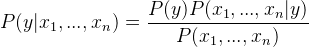Since, x1, x2, …, xn are independent of each other,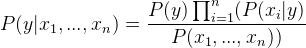Inserting proportionality by removing the P(x1, …, xn) (since, it is constant).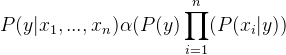Therefore, the class label is decided by,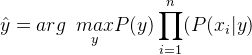P(y) is the relative frequency of class label y in the training dataset.

In case of Gaussian Naive Bayes classifier, P(xi | y) is calculated as,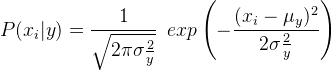`# importing necessary libraries``from` `sklearn ``import` `datasets``from` `sklearn.metrics ``import` `confusion_matrix``from` `sklearn.model_selection ``import` `train_test_split`` ` `# loading the iris dataset``iris ``=` `datasets.load_iris()`` ` `# X -> features, y -> label``X ``=` `iris.data``y ``=` `iris.target`` ` `# dividing X, y into train and test data``X_train, X_test, y_train, y_test ``=` `train_test_split(X, y, random_state ``=` `0``)`` ` `# training a Naive Bayes classifier``from` `sklearn.naive_bayes ``import` `GaussianNB``gnb ``=` `GaussianNB().fit(X_train, y_train)``gnb_predictions ``=` `gnb.predict(X_test)`` ` `# accuracy on X_test``accuracy ``=` `gnb.score(X_test, y_test)``print` `accuracy`` ` `# creating a confusion matrix``cm ``=` `confusion_matrix(y_test, gnb_predictions)`

References –

This article is contributed by Arik Pamnani. If you like GeeksforGeeks and would like to contribute, you can also write an article using contribute.geeksforgeeks.org or mail your article to contribute@geeksforgeeks.org. See your article appearing on the GeeksforGeeks main page and help other Geeks.